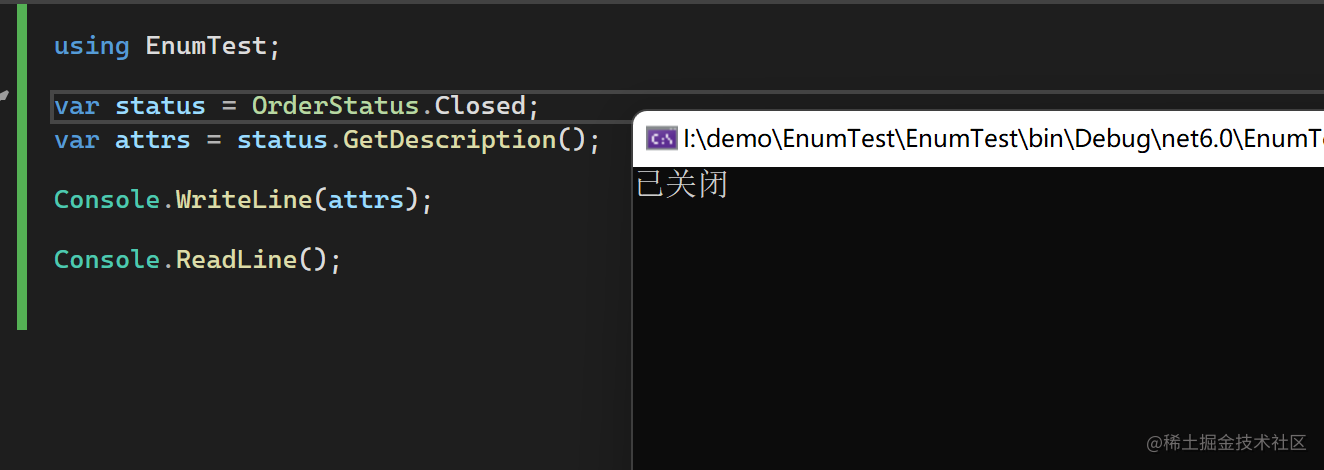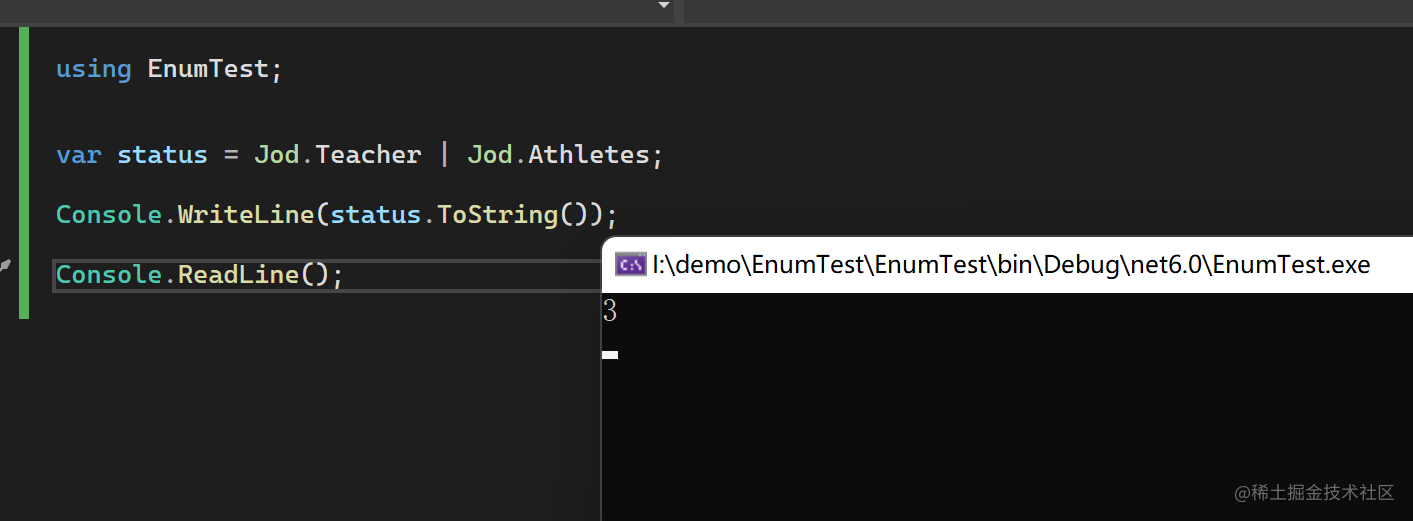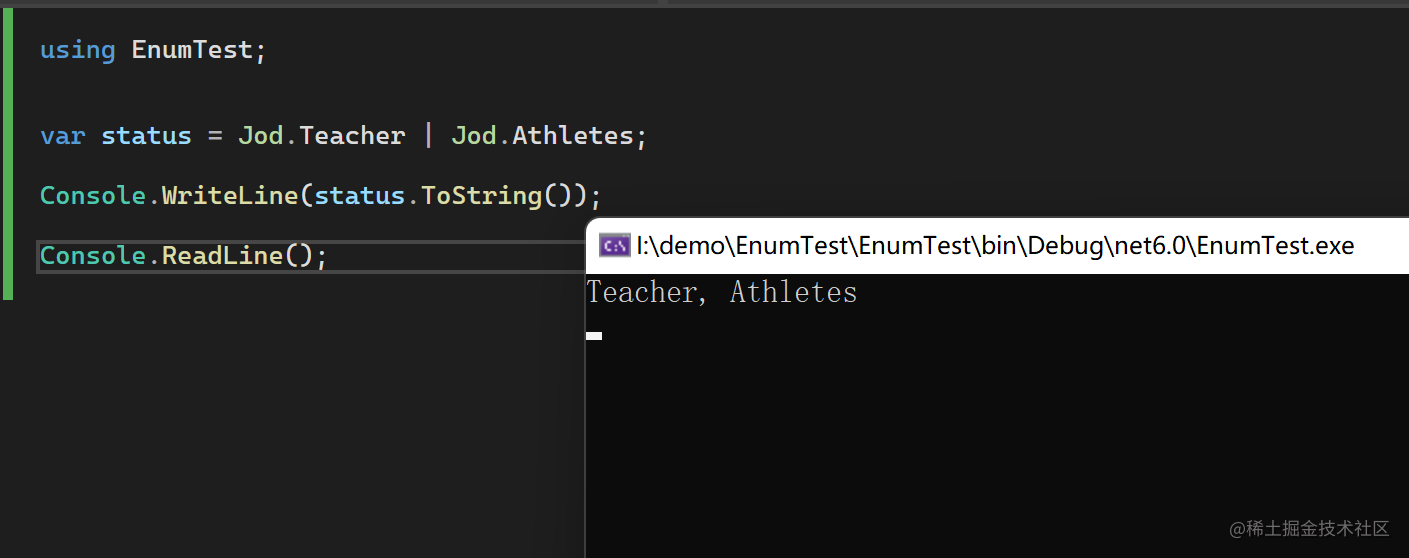# 你确定你会用Enum枚举了？# 基本使用

``````public enum OrderStatus
{
/// <summary>
/// 未支付
/// </summary>
WaitPay = 0,

/// <summary>
/// 已支付
/// </summary>
Payed = 1,

/// <summary>
/// 已退款
/// </summary>
Refund = 2,

/// <summary>
/// 已关闭
/// </summary>
Closed = 3,
}
``````

``````public enum OrderStatus: byte
{
/// <summary>
/// 未支付
/// </summary>
WaitPay = 0,

/// <summary>
/// 已支付
/// </summary>
Payed = 1,

/// <summary>
/// 已退款
/// </summary>
Refund = 2,

/// <summary>
/// 已关闭
/// </summary>
Closed = 3,
}
``````

# 搭配Description使用

``````public enum OrderStatus
{
[Description("未支付")]
WaitPay = 0,

[Description("已支付")]
Payed = 1,

[Description("已退款")]
Refund = 2,

[Description("已关闭")]
Closed = 3,
}
``````

``````public static class EnumExtensions
{
public static string GetDescription(this Enum obj)
{
object[]? array = obj.GetType().GetField(obj.ToString())?.GetCustomAttributes(typeof(DescriptionAttribute), inherit: true);
if (array != null)
{
var attr = array.FirstOrDefault(x => x is DescriptionAttribute);
if (attr != null)
{
return ((DescriptionAttribute)attr).Description;
}

}

return string.Empty;
}
}
``````# 搭配Flag属性使用

``````internal enum Jod
{
/// <summary>
/// 老师
/// </summary>
Teacher = 1,

/// <summary>
/// 运动员
/// </summary>
Athletes = 2
}
````````````[Flags]
internal enum Jod
{
/// <summary>
/// 老师
/// </summary>
Teacher = 1,

/// <summary>
/// 运动员
/// </summary>
Athletes = 2
}
``````# 位运算

``````[Flags]
internal enum Jod
{
/// <summary>
/// 老师
/// </summary>
Teacher = 1,

/// <summary>
/// 运动员
/// </summary>
Athletes = 2,

/// <summary>
/// 歌手
/// </summary>
Singer = 3,

/// <summary>
/// 舞者
/// </summary>
Dancer = 4
}
``````

``````[Flags]
internal enum Jod
{
/// <summary>
/// 老师
/// </summary>
Teacher = 1,

/// <summary>
/// 运动员
/// </summary>
Athletes = 2,

/// <summary>
/// 歌手
/// </summary>
Singer = 4,

/// <summary>
/// 舞者
/// </summary>
Dancer = 8,

Jobx = 0x10,

JobY = 0x20,

JobZ = 0x40,
...
}
``````

Teacher 1 0x1 0000 0000 0000 0000 0000 0000 0000 0001
Athletes 2 0x2 0000 0000 0000 0000 0000 0000 0000 0010
Singer 4 0x4 0000 0000 0000 0000 0000 0000 0000 0100
Dancer 8 0x8 0000 0000 0000 0000 0000 0000 0000 1000
JobX 16 0x10 0000 0000 0000 0000 0000 0000 0001 0000
JobY 32 0x20 0000 0000 0000 0000 0000 0000 0010 0000

``````// 1.基本的或运算，表示同时有多种枚举值的情况
var jobs = Jod.Teacher | Jod.Athletes;

// 2.判断某个人的职业中是否有Athletes
if ((jobs & Jod.Athletes) == Jod.Athletes)
{
// 是运动员
}

``````

# 数据库设计中的妙用

## 一般场景

merch_id 商户id long
merch_name 商户名 string
certified 已认证? int(0或1)

is_vip_merch vip商户? int(0或1)
is_defect_free 商品上架免检 int(0或1)

is_frozen 是否冻结 int(0或1)
is_mvp 是否金牌商户 int(0或1)

## 优化

merch_id 商户id long
merch_name 商户名 string
merch_flags 各种商户标识 int

``````[Flags]
public enum MerchFlags
{
/// <summary>
/// 已认证?
/// </summary>
certified = 1,
/// <summary>
/// vip商户?
/// </summary>
is_vip_merc = 2,
/// <summary>
/// 商品上架免检
/// </summary>
is_defect_free = 4,
/// <summary>
/// 是否冻结
/// </summary>
s_frozen = 8,
/// <summary>
/// 是否金牌商户
/// </summary>
is_mvp = 0x10,

// ...继续新增各种标志位
}
``````

## 思考一个问题

3 total views,  1 views today